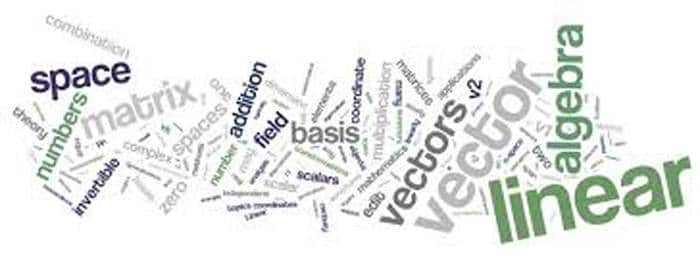Engineering Entrance Sample Papers

KEAMEE Maths Sample QuestionsQues. A tangent to the ellipse 4x2 + 9y2 = 36 is cut by the tangent at the extremities of the major axis at T and T’. The circle on TT’ as diameter passes through the point
(a) (–√5, 0)
(b) (√5 , 1)
(c) (0, 0)
(d) (3, 2)
Ans. (a)

Ques. Let S be the set of all real numbers. Then the relation R = {(a, b) : 1 + ab > 0} on S is
(a) Reflexive and symmetric but not transitive
(b) Reflexive and transitive but not symmetric
(c) Symmetric, transitive but not reflexive
(d) Reflexive, transitive and symmetric
Ans:- (a)

Ques. Two students while solving a quadratic equation in x, one copied the constant term incorrectly and got the roots 3 and 2. The other copied the constant term and coefficient of x2 correctly as –6 and 1 respectively. The correct roots are-
(a) 3, –2
(b) –3, 2
(c) –6, –1
(d) 6, –1
Ans:- (d)

Ques. A lady gives a dinner party for six guests. The number of ways in which they may be selected from among ten friends, if two of the friends will not attend the party together is
(a) 112
(b) 140
(c) 164
(d) None of these
Ans:- (b)

Ques. If (sec A + tan A) (sec B + tan B) (sec C + tan C) = (sec A – tan A) (sec B – tan B) (sec C – tan C), then each side is equal to
(a) 1
(b) – 1
(c) 0
(d) None of these
Ans:- (a,b)

Related: Maths (JEE Mains) Sample Paper

Ques. The number of integral points (integral point means both the coordinates should be integer) exactly in the interior of the triangle with vertices (0, 0), (0, 21)  and  (21, 0), is
(a) 133
(b) 190
(c) 233
(d) 105
Ans:- (b)

Ques. If the two regression coefficient between x and y are 0.8 and 0.2, then the coefficient of correlation between them is
(a) 0.4
(b) 0.6
(c) 0.3
(d) 0.5
Ans:- (a)

Ques. If a and a + h are two consecutive approximate roots of the equation f(x) = 0 as obtained by Newtons method, then h is equal to
(a) f(a)/f’(a)
(b) f’(a)/f(a)
(c) –f’(a)/f(a)
(d) –f(a)/f’(a)
Ans:- (d)

Related: EAMCET Mathematics Sample Paper

Ques. In  group G = {0, 1, 2, 3, 4, 5} under addition modulo 6, a subgroup is
(a) {0, 2, 4}
(b) {0, 1, 3}
(c) {0, 3, 5}
(d) {0, 4, 5}
Ans:- (a)

Ques. A pack of playing cards was found to contain only 51 cards. If the first 13 cards which are examined are all red, then the probability that the missing cards is black, is
(a) 1/3
(b) 2/3
(c) 1/2
(d) 1/8
Ans:- (b)

Ques. If in a triangle ABC, c = 3b and CB = 90°, then tanB equals (The symbols have their usual meanings)
(a) 2 + √3
(b) 2 – √3
(c) 3
(d) 1/3
Ans:- (d)